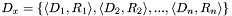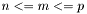#Boost C++ Libraries

...one of the most highly regarded and expertly designed C++ library projects in the world.

## Dimensional Analysis

The concept of dimensional analysis is normally presented early on in introductory physics and engineering classes as a means of determining the correctness of an equation or computation by propagating the physical measurement units of various quantities through the equation along with their numerical values. There are a number of standard unit systems in common use, the most prominent of which is the Systeme International (also known as SI or MKS (meter-kilogram-second), which was a metric predecessor to the SI system named for three of the base units on which the system is based). The SI is the only official international standard unit system and is widely utilized in science and engineering. Other common systems include the CGS (centimeter-gram-second) system and the English system still in use in some problem domains in the United States and elsewhere. In physics, there also exist a number of other systems that are in common use in specialized subdisciplines. These are collectively referred to as natural units. When quantities representing different measurables are combined, dimensional analysis provides the means of assessing the consistency of the resulting calculation. For example, the sum of two lengths is also a length, while the product of two lengths is an area, and the sum of a length and an area is undefined. The fact that the arguments to many functions (such as exp, log, etc...) must be dimensionless quantities can be easily demonstrated by examining their series expansions in the context of dimensional analysis. This library facilitates the enforcement of this type of restriction in code involving dimensioned quantities where appropriate.

In the following discussion we view dimensional analysis as an abstraction in which an arbitrary set of units obey the rules of a specific algebra. We will refer to a pair of a base dimension and a rational exponent as a fundamental dimension, and a list composed of an arbitrary number of fundamental dimensions as a composite dimension or, simply, dimension. In particular, given a set offundamental dimensions denoted byand a set ofrational exponents, any possible (composite) dimension can be written as.

Composite dimensions obey the algebraic rules for dimensional analysis. In particular, for any scalar value,, and composite dimensionsand, where, we have:Users of a dimensional analysis library should be able to specify an arbitrary list of base dimensions to produce a composite dimension. This potentially includes repeated tags. For example, it should be possible to express energy as,,, or any other permutation of mass, length, and time having aggregate exponents of 1, 2, and -2, respectively. In order to be able to perform computations on arbitrary sets of dimensions, all composite dimensions must be reducible to an unambiguous final composite dimension, which we will refer to as a reduced dimension, for which

1. fundamental dimensions are consistently ordered
2. dimensions with zero exponent are elided. Note that reduced dimensions never have more thanbase dimensions, one for each distinct fundamental dimension, but may have fewer.

In our implementation, base dimensions are associated with tag types. As we will ultimately represent composite dimensions as typelists, we must provide some mechanism for sorting base dimension tags in order to make it possible to convert an arbitrary composite dimension into a reduced dimension. For this purpose, we assign a unique integer to each base dimension. The `base_dimension` class (found in `boost/units/base_dimension.hpp`) uses the curiously recurring template pattern (CRTP) technique to ensure that ordinals specified for base dimensions are unique:

```template<class Derived, long N> struct base_dimension { ... };
```

With this, we can define the base dimensions for length, mass, and time as:

```/// base dimension of length
struct length_base_dimension : base_dimension<length_base_dimension,1> { };
/// base dimension of mass
struct mass_base_dimension : base_dimension<mass_base_dimension,2> { };
/// base dimension of time
struct time_base_dimension : base_dimension<time_base_dimension,3> { };
```

It is important to note that the choice of order is completely arbitrary as long as each tag has a unique enumerable value; non-unique ordinals are flagged as errors at compile-time. Negative ordinals are reserved for use by the library. To define composite dimensions corresponding to the base dimensions, we simply create MPL-conformant typelists of fundamental dimensions by using the `dim` class to encapsulate pairs of base dimensions and `static_rational` exponents. The `make_dimension_list` class acts as a wrapper to ensure that the resulting type is in the form of a reduced dimension:

```typedef make_dimension_list<
boost::mpl::list< dim< length_base_dimension,static_rational<1> > >
>::type   length_dimension;

typedef make_dimension_list<
boost::mpl::list< dim< mass_base_dimension,static_rational<1> > >
>::type     mass_dimension;

typedef make_dimension_list<
boost::mpl::list< dim< time_base_dimension,static_rational<1> > >
>::type     time_dimension;
```

This can also be easily accomplished using a convenience typedef provided by `base_dimension`:

```typedef length_base_dimension::dimension_type    length_dimension;
typedef mass_base_dimension::dimension_type      mass_dimension;
typedef time_base_dimension::dimension_type      time_dimension;
```

so that the above code is identical to the full typelist definition. Composite dimensions are similarly defined via a typelist:

```typedef make_dimension_list<
boost::mpl::list< dim< length_base_dimension,static_rational<2> > >
>::type   area_dimension;

typedef make_dimension_list<
boost::mpl::list< dim< mass_base_dimension,static_rational<1> >,
dim< length_base_dimension,static_rational<2> >,
dim< time_base_dimension,static_rational<-2> > >
>::type    energy_dimension;
```

A convenience class for composite dimensions with integer powers is also provided:

```typedef derived_dimension<length_base_dimension,2>::type  area_dimension;
typedef derived_dimension<mass_base_dimension,1,
length_base_dimension,2,
time_base_dimension,-2>::type   energy_dimension;
```